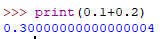# Why does print(0.1+0.2) return 0.3?

`print(0.1+0.2)` outputs `0.3`

However, `0.1+0.2` is not actually equal to 0.3, the program below proves this because it does not print ‘equal’.

``````if 0.1 + 0.2 == 0.3 then
print("equal")
end
``````

The reason for this is due to floating point approximations, but why does the print not output the ‘true’ value of `0.1+0.2` (showing the floating point error), and how can I view actual representation of `0.1+0.2`?

1 Like

If you don’t add quotations in the parenthesis then it will output the sum. Try it with parenthesis.
See “Strings v.s. Polynomials”

I would like the sum, but just the true representation of the sum (the value actually stored in the computer).

Here’s an example of the same code in python for example, the floating point approximation can clearly be seen.1 Like

It might be due to the precendence of operations as seen here.

It should then work if you change it to this:

``````if (0.1 + 0.2) == 0.3 then
print("equal")
end
``````
1 Like

I have just tested this, and can confirm that this does not work.

Yes, Roblox does tend to have that issue too (though not always shown at times). A simple way to avoid it would to set it up a bit like this:

``````local Answer = math.floor((0.1+0.2)*10)/10

print("equal")
end
``````

Or to spread out the math a little bit,

``````local Answer = 0.1 + 0.2
``````

While it is a bit unfortunate that this is a necessity here, it’s best used to avoid these sort of problems.
As long as you know for the most part how many decimal points you’d like to account for, just change the 10s to anything like 100 or 1000.

2 Likes

This is all well and good for this scenario, however my reason for this post is because I am dealing with a program that relies on infinite precision (which obviously isn’t possible in computers) and so I would like to be able to print the actual representation of my values to see where my program is going wrong. Addressing the floating point approximations in my program is not as simple as this unfortunately.

A direct answer to my question needs to address how I can view the true representation of my numbers.

Thank you for the reply though.

2 Likes

Roblox’s print function rounds numbers to the first ~11 digits, and doesn’t go beyond that, so you won’t see that level of precision unless you explicitly format it yourself:
print(string.format("%.52f",0.1 + 0.2))
`0.3000000000000000444089209850062616169452667236328125`

9 Likes

Fantastic, seems much better. Is there a reason for choosing 51 decimal places as opposed to some other number?

I edited my reply to do 52 decimal places, but anything beyond that it will just print a line of zeroes.

I’m confused here. Why would you want 0.1 + 0.2 to not equal 0.3? And, why does it really equal

this? I’ve never heard of this beforeHow is this more accurate than it being 0.3?

In an ideal world `0.1 + 0.2` would equal `0.3`, however, this is not possible for computers because they only have a limited number of bits, and so rounding errors are made.

I recommend you look up ‘floating point approximations’ and that will explain more in depth the problem.

5 Likes